# Operations with Functions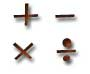We can add, subtract, multiply and divide functions! The result is a new function.

Let us try doing those operations on f(x) and g(x):(f+g)(x) = f(x) + g(x)

Note: we put the f+g inside () to show they both work on x.

### Example: f(x) = 2x+3 and g(x) = x2

(f+g)(x) = (2x+3) + (x2) = x2+2x+3

Sometimes we may need to combine like terms:

### Example: v(x) = 5x+1, w(x) = 3x-2

(v+w)(x) = (5x+1) + (3x-2) = 8x-1

The only other thing to worry about is the Domain (the set of numbers that go into the function), but I will talk about that later!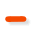### Subtraction

We can also subtract two functions:

(f-g)(x) = f(x) − g(x)

### Example: f(x) = 2x+3 and g(x) = x2

(f-g)(x) = (2x+3) − (x2)### Multiplication

We can multiply two functions:

(f·g)(x) = f(x) · g(x)

### Example: f(x) = 2x+3 and g(x) = x2

(f·g)(x) = (2x+3)(x2) = 2x3 + 3x2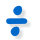### Division

And we can divide two functions:

(f/g)(x) = f(x) / g(x)

### Example: f(x) = 2x+3 and g(x) = x2

(f/g)(x) = (2x+3)/x2

## Function Composition

 There is another special operation called Function Composition, read that page to find out more! (g º f)(x)

## Domains

It has been very easy so far, but now we must consider the Domains of the functions.The domain is the set of all the values that go into a function.

The function must work for all values we give it, so it is up to us to make sure we get the domain correct!

### Example: the domain for √x (the square root of x)

We can't have the square root of a negative number (unless we use imaginary numbers, but we aren't doing that here), so we must exclude negative numbers:

The Domain of √x is all non-negative Real Numbers

On the Number Line it looks like: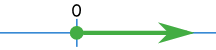Using set-builder notation it is written:

{ x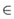| x ≥ 0}

"the set of all x's that are a member of the Real Numbers,
such that x is greater than or equal to zero
"

Or using interval notation it is:

[0,+∞)

It is important to get the Domain right, or we will get bad results!

So how do we work out the new domain after doing an operation?

## How to Work Out the New Domain

When we do operations on functions, we end up with the restrictions of both.It is like cooking for friends:

• one can't eat peanuts,
• the other can't eat dairy food.

So what we cook can't have peanuts and also can't have dairy products.

### Example: f(x)=√x and g(x)=√(3−x)

The domain for f(x)=√x is from 0 onwards:The domain for g(x)=√(3−x) is up to and including 3: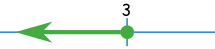So the new domain (after adding or whatever) is from 0 to 3: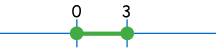If we choose any other value, then one or the other part of the new function won't work.

In other words we want to find where the two domains intersect.

Note: we can put this whole idea into one line using Set Builder Notation:

Dom(f+g) = { x| xDom(f) and xDom(g) }

Which says "the domain of f plus g is the set of all Real Numbers that are in the domain of f AND in the domain of g"

The same rule applies when we add, subtract, multiply or divide, except divide has one extra rule.

## An Extra Rule for Division

There is an extra rule for division:

As well as restricting the domain as above, when we divide:

(f/g)(x) = f(x) / g(x)

we must also make sure that g(x) is not equal to zero (so we don't divide by zero).

Here is an example:

### Example: f(x)=√x and g(x)=√(3−x)

(f/g)(x) = √x / √(3−x)

1. The domain for f(x)=√x is from 0 onwards:2. The domain for g(x)=√(3−x) is up to and including 3:3. AND √(3−x) cannot be zero, so x cannot be 3: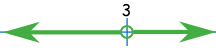(Notice the open circle at 3, which means not including 3)

So all together we end up with: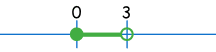## Summary

• To add, subtract, multiply or divide functions just do as the operation says.
• The domain of the new function will have the restrictions of both functions that made it.
• Divide has the extra rule that the function we are dividing by cannot be zero.